# Plotting a point in quadrant 1: Mixed number coordinates Online Quiz

Following quiz provides Multiple Choice Questions (MCQs) related to Plotting a point in quadrant 1: Mixed number coordinates. You will have to read all the given answers and click over the correct answer. If you are not sure about the answer then you can check the answer using Show Answer button. You can use Next Quiz button to check new set of questions in the quiz.### Explanation

Step 1 − The x coordinate and y coordinate of the point are 3 and$1\frac{3}{4}$ respectively and the point lies in the quadrant 1.

Step 2 − Plotting the point $(3, 1\frac{3}{4})$ in the quadrant 1 we get.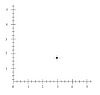### Explanation

Step 1 − The x coordinate and y coordinate of the point are 5 and $2\frac{2}{3}$ respectively and the point lies in the quadrant 1.

Step 2 − Plotting the point $(5, 2\frac{2}{3})$ in the quadrant 1 we get.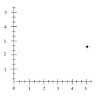### Explanation

Step 1 − The x coordinate and y coordinate of the point are $3\frac{3}{4}$ and 2 respectively and the point lies in the quadrant 1.

Step 2 − Plotting the point $(3\frac{3}{4}, 2)$ the quadrant 1 we get.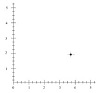### Explanation

Step 1 − The x coordinate and y coordinate of the point are 5 and $1\frac{1}{3}$ respectively and the point lies in the quadrant 1.

Step 2 − Plotting the point $(5, 1\frac{1}{3})$ in the quadrant 1 we get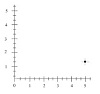### Explanation

Step 1 − The x coordinate and y coordinate of the point are 0 and $2\frac{1}{2}$ respectively and the point lies on the positive y axis.

Step 2 − Plotting the point $(0, 2\frac{1}{2})$ in the quadrant 1 we get### Explanation

Step 1 − The x coordinate and y coordinate of the point are 4 and $1\frac{2}{3}$ respectively and the point lies in the quadrant 1.

Step 2 − Plotting the point $(4, 1\frac{2}{3})$ in the quadrant 1 we get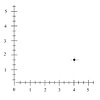### Explanation

Step 1 − The x coordinate and y coordinate of the point are 4 and $1\frac{1}{2}$ respectively and the point lies in the quadrant 1.

Step 2 − Plotting the point $(1\frac{2}{3}, 5)$ in the quadrant 1 we get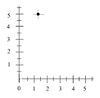### Explanation

Step 1 − The x coordinate and y coordinate of the point are $\frac{1}{3}$ and 3 respectively and the point lies in the quadrant 1.

Step 2 − Plotting the point $(\frac{1}{3}, 3)$ in the quadrant 1 we get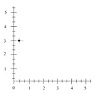### Explanation

Step 1 − The x coordinate and y coordinate of the point are $3\frac{3}{4}$ and 0 respectively and the point lies the positive x axis.

Step 2 − Plotting the point $(3\frac{3}{4}, 0)$ in the quadrant 1 we get### Explanation

Step 1 − The x coordinate and y coordinate of the point are 0 and $2\frac{3}{4}$ respectively and the point lies in the quadrant 1.

Step 2 − Plotting the point $(4, 2\frac{3}{4})$ in the quadrant 1 we get